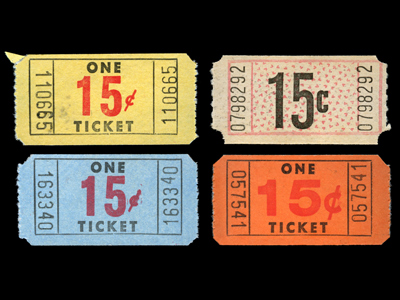The 15 times tables is one of the easier tables!

# The 15 Times Table

Memorising as many times tables as possible is a good idea if you want to do well in maths, and KS3 Maths is no different. Usually, people learn the times tables up to 12 but we thought you'd like a bit more of a challenge. So we're going to test your times table knowledge all the way up to the 20 times table. In this quiz we look at the 15 times table.

165, 225 and 285 are multiples of 15 and so they are all found in the 15 times table. 15 is a triangular number and one which we come across quite often. Each player has 15 counters at the start of a game of backgammon and there are 15 red balls in a game of snooker. 15 is also an odd number. The Roman natural historian and philosopher, Pliny the Elder, said "Why do we believe that in all matters the odd numbers are more powerful?"

It's all about practise when it comes to memorising the times tables. Have a go at this quiz and see how well you know the 15 times table.

1.
What is 18 x 15
270
280
265
264
20 x 15 = 300 and 2 x 15 = 30 so 300 - 30 = 15 x 18
2.
What is 15 x 15
235
226
234
225
15 is the square root of 225
3.
What is 11 x 15
174
168
169
165
10 x 15 = 150 and 150 + 15 = 165
4.
What is 19 x 15
280
276
285
281
20 x 15 = 300 and 300 - 15 = 285
5.
What is 5 x 15
77
66
75
73
10 x 15 = 150 so halve 150 to find the answer
6.
What is 9 x 15
126
135
125
143
10 x 15 = 150 and 150 - 15 = 135
7.
What is 20 x 15
300
305
315
291
2 x 15 = 30 so 20 x 15 = 300
8.
What is 17 x 15
260
258
250
255
In Roman numerals 255 is written as CCLV
9.
What is 10 x 15
156
155
149
150
To multiply any number by 10 simply put a zero on its end
10.
What is 6 x 15
89
88
90
96
5 x 15 = 75 and 75 + 15 = 90
Author:  Frank Evans